Pseudo-Riemannian manifolds in SageMath¶

The Schwarzschild spacetime example¶

This notebook demonstrates some SageMath tools for pseudo-Riemannian geometry, developed through the SageManifolds project, by these authors.

This notebook requires a version of SageMath at least equal to 9.0:

In :
version()
Out:
'SageMath version 9.2, Release Date: 2020-10-24'

First we set up the notebook to display outputs via LaTeX rendering:

In :
%display latex

Since some computations are quite heavy, we ask for running them in parallel on 8 threads:

In :
Parallelism().set(nproc=8)

We introduce the Schwarzschild spacetime, which is the spacetime of a static black hole in general relativity, as a 4-dimensional Lorentzian manifold $M$:

In :
M = Manifold(4, 'M', structure='Lorentzian')
M
Out:
In :
print(M)
4-dimensional Lorentzian manifold M

$M$ is in the category of smooth manifolds over the real field:

In :
M.category()
Out:
In :
M.base_field()
Out:

At the moment, the real field is modeled by 53-bit floating-point approximations, but this plays no role in the manifold implementation:

In :
print(M.base_field())
Real Field with 53 bits of precision

Coordinate charts¶

The function Manifold generates a manifold with no-predefined coordinate chart, so that the manifold (user) atlas is empty:

In :
M.atlas()
Out:

We introduce the standard Schwarzchild-Droste coordinates $(t,r,\theta,\phi)$ on $M$, via the method chart:

In :
SD.<t, r, th, ph> = M.chart(r"t r:(0,+oo) th:(0,pi):\theta ph:(0,2*pi):\phi:periodic")

Note that the argument of chart() is a raw string (hence the prefix r in front of it), which defines the range of each coordinate, if different from $(-\infty, +\infty)$, as well as its LaTeX symbol, if different from the Python symbol to denote the coordinate. The Python variables for each coordinate are declared within the <...> operator on the left-hand side, SD denoting the Python variable chosen for the coordinate chart.

In :
SD
Out:
In :
print(SD)
Chart (M, (t, r, th, ph))
In :
SD.coord_range()
Out:

Thanks to the SageMath operator <...> used in the chart declaration, the coordinates are immediately available:

In :
th
Out:

They are SageMath's symbolic expressions:

In :
th.parent()
Out:

They are also accessible as items of the chart:

In :
SD, SD
Out:
In :
SD[:]
Out:

The manifold (user) atlas is no longer empty:

In :
M.atlas()
Out:

Let us introduce a second chart on the manifold, that of Eddington-Finkelstein coordinates $(T,r,\theta,\phi)$:

In :
EF.<T, r, th, ph> = M.chart(r"T r:(0,+oo) th:(0,pi):\theta ph:(0,2*pi):\phi:periodic")
EF
Out:

The transition map from Schwarzschild-Droste coordinates (chart SD) to Eddington-Finkelstein ones (chart EF) depends on a parameter $m$, the mass of the Schwarzschild black hole:

In :
m = var('m')
assume(m > 0)

We provide the explicit coordinate transformation via the method transition_map:

In :
SD_to_EF = SD.transition_map(EF, [t +2*m*ln(abs(r/(2*m)-1)), r, th, ph])
SD_to_EF.display()
Out:
In :
SD_to_EF.inverse().display()
Out:

There are now two charts in the manifold atlas:

In :
M.atlas()
Out:

One of them is the so-called default chart: it is the chart used by any function that requires a chart as argument and none is provided by the user. At this stage, the default chart is the first chart defined on the manifold, but this can be changed by the manifold method set_default_chart.

In :
M.default_chart()
Out:

One can plot the SD chart in terms of EF one:

In :
plot1 = SD.plot(EF, ranges={t:(0, 8), r:(2.1, 10)}, fixed_coords={th:pi/2, ph:0},
ambient_coords=(r,T), style={t:'--', r:'-'}, parameters={m: 1}) \
+ SD.plot(EF, ranges={t:(0, 8), r:(0.1, 1.9)}, fixed_coords={th:pi/2, ph:0},
ambient_coords=(r,T), number_values={t: 9, r: 3},
style={t:'--', r:'-'}, parameters={m: 1})
plot1
Out: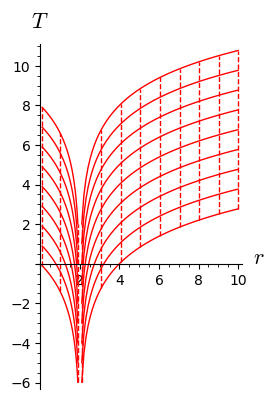Manifold points¶

To create a point on $M$, we use SageMath's parent/element syntax, i.e. the call operator M(...) acting on the parent M, with the point's coordinates in some chart as argument:

In :
p = M((m, 8*m, pi/2, 0), name='p')
print(p)
Point p on the 4-dimensional Lorentzian manifold M

Since the chart has not been specified, the default chart (i.e. SD) is meant:

In :
SD(p)
Out:

Thanks to the transition map declared above, the coordinates of $p$ in the Eddington-Finkelstein chart can computed:

In :
EF(p)
Out:

Manifold points have a plot method:

In :
plot1 += p.plot(EF, color='blue', ambient_coords=(r,T),
parameters={m: 1}, label_offset=0.4, fontsize=14)
plot1
Out: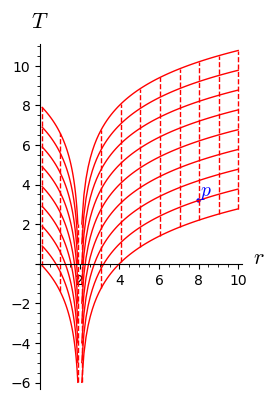Vector fields¶

When a chart is declared, the manifold is automatically endowed with some vector fields, those of the coordinate vector frame:

In :
SD.frame()
Out:
In :
EF.frame()
Out:
In :
M.frames()
Out:

As for charts, there is a default frame, i.e. a vector frame that is used by default in functions having a vector frame in their arguments. The default frame can be changed by the method set_default_frame.

In :
M.default_frame()
Out:

The first vector of the Schwarzschild-Droste coordinate frame:

In :
vt = SD.frame()
vt
Out:
In :
vt.display()
Out:
In :
vt.display(EF.frame())
Out:

The second vector of the Schwarzschild-Droste coordinate frame:

In :
vr = SD.frame()
vr
Out:
In :
vr.display(EF.frame())
Out:

Creating a vector field from scratch, by providing its components with rest to a given vector frame:

In :
k = M.vector_field(1, -1, 0, 0, frame=EF.frame(), name='k')
k.display()
Out:
In :
k.display(EF.frame())
Out:

Plot with respect to Schwarzschild-Droste coordinates (default chart):

In :
k.plot(ambient_coords=(r,t), fixed_coords={th: pi/2, ph: 0},
ranges={t: (0, 6), r: (0.1, 6)}, number_values=9,
parameters={m: 1}, color='green', scale=0.3)
Out: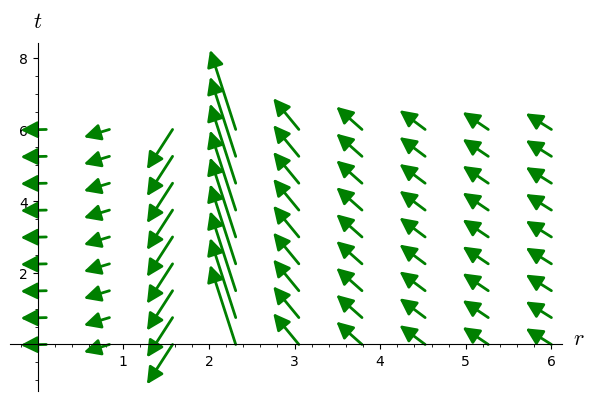Plot with respect to Eddington-Finkelstein coordinates:

In :
k.plot(chart=EF, ambient_coords=(r,T), chart_domain=EF,
fixed_coords={th: pi/2, ph: 0}, ranges={T: (0, 8), r: (0.1, 10)},
number_values=5, parameters={m: 1}, color='green')
Out: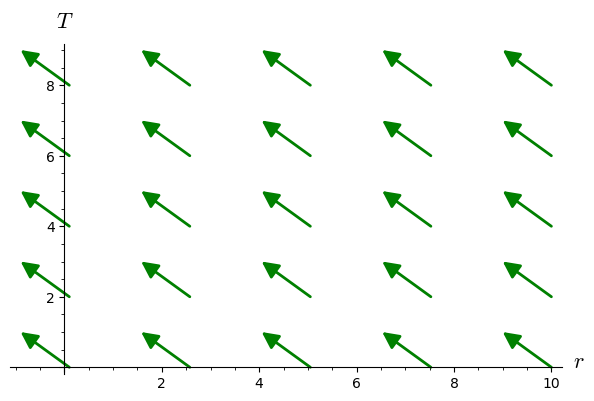Vector fields as sections of the tangent bundle¶

In :
TM = M.tangent_bundle()
print(TM)
Tangent bundle TM over the 4-dimensional Lorentzian manifold M
In :
k1 = TM.section({EF.frame(): [1, -1, 0, 0]})
print(k1)
Vector field on the 4-dimensional Lorentzian manifold M
In :
k1 == k
Out:

The set of all vector fields on $M$ as a $C^\infty(M)$-module:¶

In :
XM = M.vector_field_module()
print(XM)
XM
Free module X(M) of vector fields on the 4-dimensional Lorentzian manifold M
Out:
In :
XM.base_ring()
Out:
In :
print(XM.base_ring())
Algebra of differentiable scalar fields on the 4-dimensional Lorentzian manifold M
In :
XM.base_ring().an_element().display()
Out:

Vectors at a point¶

The value of a vector field, and more generally of any tensor field, at a given point is obtained by the method at:

In :
kp = k.at(p)
print(kp)
Tangent vector k at Point p on the 4-dimensional Lorentzian manifold M
In :
kp.display()
Out:

The parent of kp is the tangent space at p:

In :
kp.parent()
Out:
In :
print(kp.parent())
Tangent space at Point p on the 4-dimensional Lorentzian manifold M

It is accessible from the manifold via the method tangent_space:

In :
kp.parent() is M.tangent_space(p)
Out:

$T_p M$ is the fiber over $p$ in the tangent bundle $TM$:

In :
kp.parent() is TM.fiber(p)
Out:

Tangent vectors have a method plot:

In :
plot1 += kp.plot(EF, color='green', ambient_coords=(r,T),
parameters={m: 1}, scale=2, label_offset=0.5,
fontsize=16)
plot1
Out: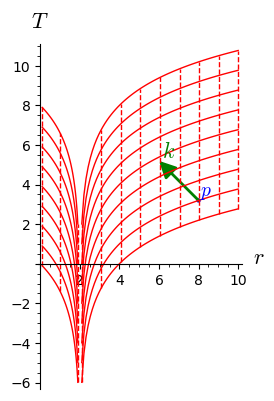Vector field defined on an open subset¶

Let us introduce the exterior $E$ of the black hole as an open subset of $M$:

In :
E = M.open_subset('E', coord_def = {SD: r>2*m})
In :
SD.restrict(E).coord_range()
Out:
In :
p in E
Out:
In :
u = E.vector_field(name='u')
u = 1/sqrt(1-2*m/r)
u.display()
Out:
In :
SD_to_EF.restrict(E)
Out:
In :
E.atlas()
Out:
In :
u.display(EF.frame().restrict(E))
Out:
In :
u.plot(ambient_coords=(r, t), fixed_coords={th: pi/2, ph: 0},
ranges={t: (0, 6), r: (2.1, 6)}, number_values=5,
parameters={m: 1}, scale=0.3)
Out: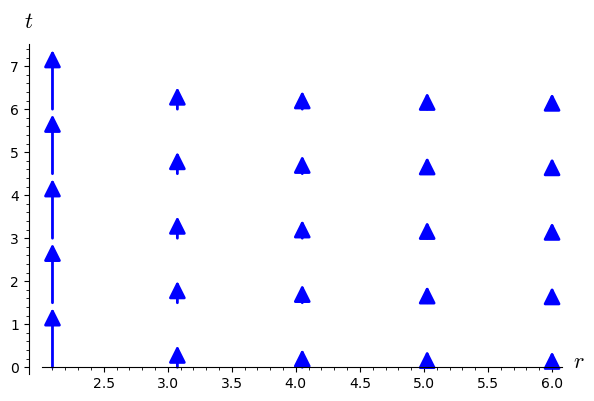In :
u.at(p).display()
Out:

Metric tensor¶

We define next the metric tensor $g$ from its non-vanishing components in the manifold's default frame, namely the coordinate frame associated to Schwarzschild-Droste coordinate:

In :
g = M.metric()
g[0, 0] = - (1 - 2*m/r)
g[1, 1] = 1/(1 - 2*m/r)
g[2, 2] = r^2
g[3, 3] = r^2*sin(th)^2
In :
g.display()
Out:
In :
SD.coframe()
Out:
In :
g[:]
Out:
In :
g[1,1]
Out:

$g_{rr}$ is diverging at $r=2m$: this is a singularity of the Schwarszchild-Droste coordinates.

In :
g.display_comp()
Out:

The components of $g$ with respect to the Eddington-Finkelstein frame are evaluated via the methods comp or display:

In :
g.display(EF.frame())
Out:
In :
g[EF.frame(),:]
Out:

Note that these components are regular at $r=2m$, contrary to the components in Schwarzschild-Droste coordinates.

The metric tensor is a twice-covariant tensor (actually a field of symmetric bilinear forms):

In :
g.tensor_type()
Out:

It can be thus be applied to a pair of vector fields, for instance $u$ and $k$:

In :
s = g(u, k)
s
Out:
In :
print(s)
Scalar field g(u,k) on the Open subset E of the 4-dimensional Lorentzian manifold M
In :
s.display()
Out:
In :
s.expr()
Out:
In :
g(k, k).display()
Out:

$u$ is a unit timelike vector:

In :
g(u, u).display()
Out:

Scalar products returned by the method dot are actually those formed with $g$:

In :
u.dot(u)
Out:
In :
u.dot(u).display()
Out:

Levi-Civita connection¶

In :
nabla = g.connection()
print(nabla)
Levi-Civita connection nabla_g associated with the Lorentzian metric g on the 4-dimensional Lorentzian manifold M
In :
nabla.display()
Out:
In :
Parallelism().set(nproc=1)
In :
Dk = nabla(k)
Dk
Out:
In :
print(Dk)
Tensor field nabla_g(k) of type (1,1) on the 4-dimensional Lorentzian manifold M
In :
Dk.display()
Out:
In :
Dk.display_comp()
Out:

The acceleration $\nabla_k k$ of the vector field $k$:

In :
Ak = Dk.contract(k)
print(Ak)
Vector field on the 4-dimensional Lorentzian manifold M
In :
Ak.display()
Out:

$k$ is thus geodesic vector. The field lines of $k$ are actually the ingoing radial null geodesics of Schwarzschild spacetime.

Instead of the method contract, one can use index notations to compute $\nabla_k k$ as $k^b \nabla_b k^a$:

In :
Ak == Dk['^a_b']*k['^b']
Out:
In :
Parallelism().set(nproc=8)

The acceleration $\nabla_u u$ of the vector field $u$:

In :
Au = nabla(u).contract(u)
Au.display()
Out:

$\nabla g$ is identically zero, since the connection $\nabla$ is compatible with $g$:

In :
Dg = nabla(g)
print(Dg)
Tensor field nabla_g(g) of type (0,3) on the 4-dimensional Lorentzian manifold M
In :
Dg.display()
Out:

Curvature¶

The Riemann curvature tensor is computed as

In :
Riem = g.riemann()
print(Riem)
Tensor field Riem(g) of type (1,3) on the 4-dimensional Lorentzian manifold M
In :
Riem.display_comp(only_nonredundant=True)
Out:

The component $\mathrm{Riem}(g)^t_{\ \, rtr} = \mathrm{Riem}(g)^0_{\ \, 101}$ is returned by

In :
Riem[0,1,0,1]
Out:
In :
Riem.display_comp(EF.frame(), only_nonredundant=True)
Out:

The Kretschmann scalar is the "square" of the Riemann tensor defined by $$K = R_{abcd} \, R^{abcd}, \qquad R := \mathrm{Riem}(g)$$ To compute it, we must first form the tensor fields whose components are $R_{abcd}$ and $R^{abcd}$. They are obtained by respectively lowering and raising the indices of the components $R^a_{\ \, bcd}$ of the Riemann tensor, via the metric $g$. These two operations are performed by the methods down() and up(). The contraction is performed by summation on repeated indices, using LaTeX notations:

In :
K = Riem.down(g)['_{abcd}'] * Riem.up(g)['^{abcd}']
print(K)
K.display()
Scalar field on the 4-dimensional Lorentzian manifold M
Out:
In :
K.expr()
Out:

Since $\lim_{r\to 0} K = +\infty$, we may say that $r=0$ is a curvature singularity of Schwarzschild spacetime.

Ricci tensor¶

In :
Ric = g.ricci()
print(Ric)
Field of symmetric bilinear forms Ric(g) on the 4-dimensional Lorentzian manifold M

We check that the Schwarzschild metric is a solution of the vacuum Einstein equation:

In :
Ric.display()
Out:

Geodesics¶

First, for graphical purposes, we introduce the Euclidean space $\mathbb{E}^3$ and some map $M\to \mathbb{E}^3$:

In :
E3.<x,y,z> = EuclideanSpace()
X3 = E3.cartesian_coordinates()
to_E3 = M.diff_map(E3, {(SD, X3):
[r*sin(th)*cos(ph), r*sin(th)*sin(ph), r*cos(th)]})
to_E3.display()
Out:

A timelike geodesic¶

Let us consider the geodesic starting at point $p$ and having the following tangent vector at $p$ (note that the tangent vector $v_0$ is constructed by means of the call operator () acting of the parent, which is the tangent space to $M$ at $p$):

In :
v0 = M.tangent_space(p)((1.3, 0, 0, 0.064/m), name='v_0')
v0.display()
Out:

We declare the geodesic with such initial conditions, denoting by $s$ the affine parameter (proper time), with $(s_{\rm min}, s_{\rm max})=(0, 1500\,m)$:

In :
s = var('s')
geod = M.integrated_geodesic(g, (s, 0, 2000), v0)
geod
Out:

Note that the initial point p is not explicitely passed in the argument list of integrated_geodesic, because this piece of information is contained in v0:

In :
p is v0.parent().base_point()
Out:
In :
sol = geod.solve(parameters_values={m: 1})  # numerical integration
interp = geod.interpolate()                 # interpolation of the solution for the plot
In :
geod.plot_integrated(chart=X3, mapping=to_E3, plot_points=1000,
thickness=2, label_axes=False) \
+ p.plot(chart=X3, mapping=to_E3, size=4, parameters={m: 1}) \
+ sphere(size=2, color='grey')
Out:

A 2D view by suppressing $z$ from the ambient coordinates:

In :
bh_plot = circle((0, 0), 2, edgecolor='black', fill=True, facecolor='grey', alpha=0.5)
geod.plot_integrated(chart=X3, mapping=to_E3, ambient_coords=(x,y), plot_points=1000,
thickness=2) \
+ p.plot(chart=X3, mapping=to_E3, ambient_coords=(x,y), size=4, parameters={m: 1}) \
+ bh_plot
Out: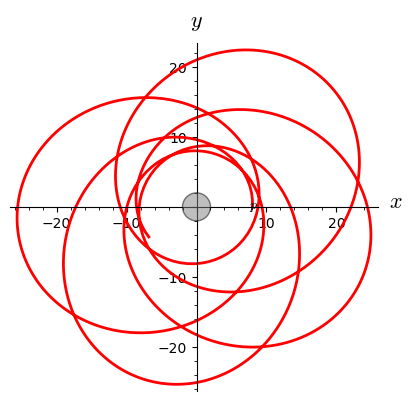Null geodesics¶

Let us consider a null geodesique $\mathscr{L}$ in the equatorial plane ($\theta = \pi/2$). The null vector $v$ tangent to $\mathscr{L}$ and associated to some affine parameter $\lambda$ is given by the following first integrals of the geodesic equation: $$v^t = \frac{\mathrm{d}t}{\mathrm{d}\lambda} = \left(1 - \frac{2m}{r} \right)^{-1}$$ $$v^r = \frac{\mathrm{d}r}{\mathrm{d}\lambda} = \pm \sqrt{1 - \frac{b^2}{r^2} \left(1 - \frac{2m}{r} \right) }$$ $$v^\theta = \frac{\mathrm{d}\theta}{\mathrm{d}\lambda} = 0$$ $$v^\varphi = \frac{\mathrm{d}\varphi}{\mathrm{d}\lambda} = \frac{b}{r^2}$$ where the constant $b$ is related to the conserved energy $E$ and conserved angular momentum $L$ along the geodesic by $$b = \frac{L}{E} .$$ For a geodesic arising from infinity, $b$ is the impact parameter.

To set up the initial vector for the computation of a null geodesic, let us define a function that takes $b$ and some initial radius $r_0$ as input and returns the initial vector $v_0$. We take advantage that SageMath is built atop Python to construct this function as a pure Python function:

In :
def initial_vector(r0, b, phi0=0, inward=True):
r"""
Evaluate the initial tangent vector along a null geodesic.

INPUT:

- r0: radial SD coordinate of the initial point
- b: impact parameter
- phi0: azimuthal SD coordinate of the initial point (default: 0)
- inward: determines whether the geodesic has initially v^r < 0 (default: True)

"""
vt0 = 1/(1 - 2*m/r0)
vr0 = sqrt(1 - b^2/r0^2*(1 - 2*m/r0))
if inward:
vr0 = - vr0
vth0 = 0
vph0 = b / r0^2
p0 = M((0, r0, pi/2, phi0), name='p_0')  # initial point
return M.tangent_space(p0)((vt0, vr0, vth0, vph0), name='v_0')

Let us use this function to construct the initial vector for $r_0 = 10m$ and $b=7m$:

In :
v0 = initial_vector(10*m, 7*m)
v0.display()
Out:

Let us check that $v_0$ is a null vector:

In :
p0 = v0.parent().base_point()
g.at(p0)(v0, v0)
Out:
In :
geod = M.integrated_geodesic(g, (s, 0, 40), v0)
sol = geod.solve(step=0.01, parameters_values={m: 1})
interp = geod.interpolate()
plot2 = geod.plot_integrated(chart=X3, mapping=to_E3, ambient_coords=(x,y),
plot_points=500, color='green', thickness=1.5, display_tangent=True,
color_tangent='green', plot_points_tangent=4, scale=1)
plot2 += bh_plot
plot2
Out: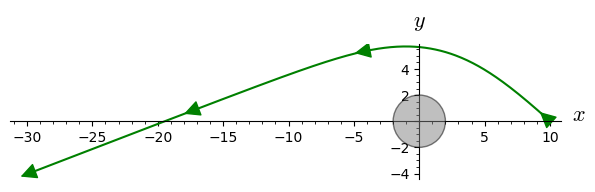A null geodesic plunging into the black hole:

In :
v0 = initial_vector(10*m, 5*m)
v0.display()
Out:
In :
geod = M.integrated_geodesic(g, (s, 0, 13), v0)
sol = geod.solve(step=0.01, parameters_values={m: 1})
interp = geod.interpolate()
plot2 += geod.plot_integrated(chart=X3, mapping=to_E3, ambient_coords=(x,y),
plot_points=500, color='green', thickness=1.5, display_tangent=True,
color_tangent='green', plot_points_tangent=3, scale=0.2)
plot2
Out: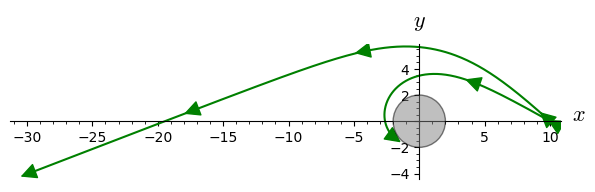The photon orbit¶

The photon orbit corresponds to $r_0=3m$ with the following critical value of the impact parameter: $$b_{\rm c} = 3\sqrt{3}\, m$$

In :
bc = 3*sqrt(3)*m
n(bc/m)
Out:
In :
v0 = initial_vector(3*m, bc)
v0.display()
Out:
In :
geod = M.integrated_geodesic(g, (s, 0, 100), v0)
sol = geod.solve(step=0.01, parameters_values={m: 1})
interp = geod.interpolate()
plot2 += geod.plot_integrated(chart=X3, mapping=to_E3, ambient_coords=(x,y),
plot_points=500, color='green', thickness=1.5)
plot2
Out: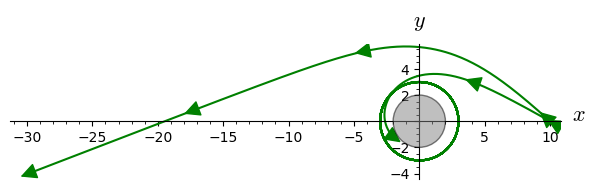A geodesic with $b$ close to $b_{\rm c}$ wraps around the circular orbit:

In :
v0 = initial_vector(10*m, 5.2025*m)
In :
geod = M.integrated_geodesic(g, (s, 0, 40), v0)
sol = geod.solve(step=0.01, parameters_values={m: 1})
interp = geod.interpolate()
plot2 += geod.plot_integrated(chart=X3, mapping=to_E3, ambient_coords=(x,y),
plot_points=500, color='orange', thickness=1.5,
display_tangent=True, color_tangent='orange',
plot_points_tangent=4, scale=1)
plot2
Out: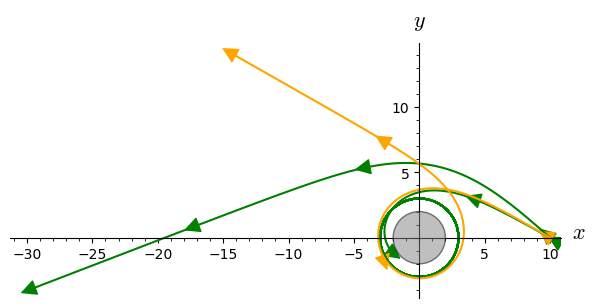Using SymPy as the symbolic backend¶

By default, the symbolic backend used in tensor calculus is SageMath's one (Pynac + Maxima), implemented via the symbolic ring SR. We can choose to use SymPy instead:

In :
M.set_calculus_method('sympy')
In :
v = 2*k
In :
v.display()
Out:
In :
v
Out:
In :
v.expr()
Out:
In :
type(v.expr())
Out:
In :
M.set_calculus_method('SR')
In :
type(v.expr())
Out:

Going further¶

Visit the SageManifolds examples.